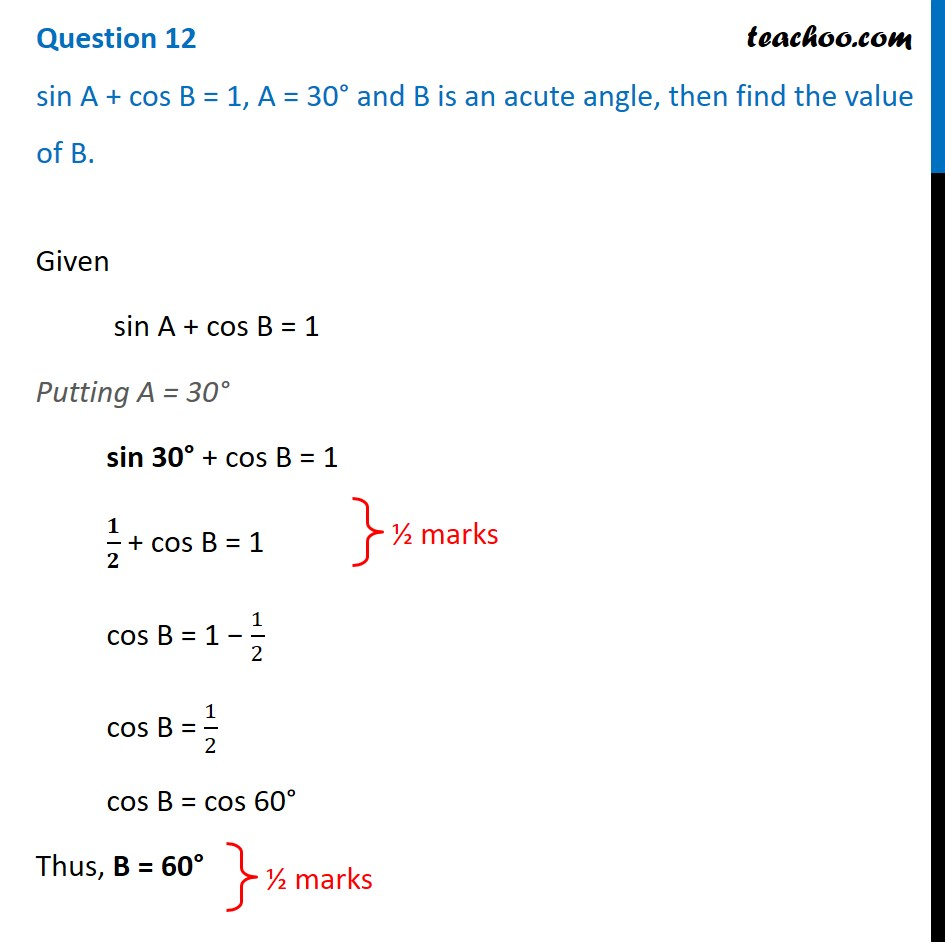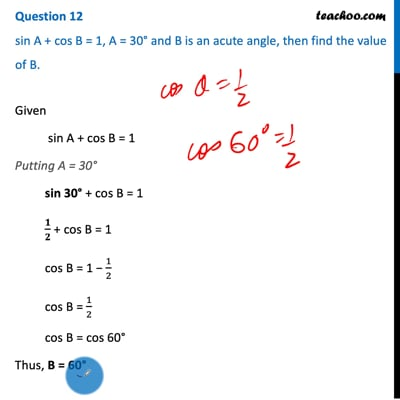CBSE Class 10 Sample Paper for 2021 Boards - Maths Standard

Class 10
Solutions of Sample Papers for Class 10 Boards

## Sin A + Cos B = 1, A = 30° and B is an acute angle, then find the value of B.This video is only available for Teachoo black users

Introducing your new favourite teacher - Teachoo Black, at only ₹83 per month

### Transcript

Question 12 sin A + cos B = 1, A = 30° and B is an acute angle, then find the value of B.Given sin A + cos B = 1 Putting A = 30° sin 30° + cos B = 1 𝟏/𝟐 + cos B = 1 cos B = 1 − 1/2 cos B = 1/2 cos B = cos 60° Thus, B = 60°